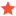• 0 Vote(s) - 0 Average
• 1
• 2
• 3
• 4
• 5
 Rotation Matrix using API?mnurpeiissovJunior MemberPosts: 22 Threads: 4 Joined: Jan 2019 Reputation: 2 02-08-2019, 07:28 AM Hello,  Is there a way to extract rotation matrix? The Mat which is returned when calling robot.SolveFK contains the information about the pose of the robot where the first three elements of last column represent the X,Y,Z positions. Is the 3x3  (first three cols and first three rows) of Mat is rotation matrix? If I use the Pose_2_TxyzRxyz and then construct the rotation matrix using Euler angle convention rotz*roty*rotx  (I am not sure if this method is right), I end up with different result than 3x3 of Mat. I would appreciate any help.  Best Regards Find ReplyAlbertAdministratorPosts: 755 Threads: 1 Joined: Apr 2018 Reputation: 35 02-08-2019, 07:18 PM This is not correct. Calling the following function:     pose = TxyzRxyz_2_Pose([x,y,z,rx,ry,rz])    Where x,y,z is the translation in mm and rx,ry,rz is the rotation in radians. It ss the same as doing the following:     pose = transl(x,y,z)*rotx(rx)*roty(ry)*rotz(rz) And you can revert this operation doing:     x,y,z,rx,ry,rz = Pose_2_TxyzRxyz(pose) More information here: https://robodk.com/doc/en/PythonAPI/robo...2_TxyzRxyz If you are using the Python API and you only want the rotation matrix you can do: rotation = pose[0:3,0:3] Another tip: You can change the coordinate system of a point the following way: point_ref1 = [x,y,z,1] point_ref2 = pose * point_ref1 You can change the coordinate system of a vector the following way: vector_ref1 = [u,v,w] vector_ref2 = pose * vector_ref1 Find ReplymnurpeiissovJunior MemberPosts: 22 Threads: 4 Joined: Jan 2019 Reputation: 2 02-09-2019, 03:29 PM (This post was last modified: 02-09-2019, 03:32 PM by mnurpeiissov.) Thank you for your reply.  I have constructed transformation matrix using DH table and calculated it using MATLAB. I came up with different rotation and translation when all joints are zero. When all the joints set to 0, robodk shows that translation along X,Y,Z are [-817 , -191, -4.45] respectively. The calculated ones are [-817, -9.53, -5.33]. So we have different Y and Z translations. The rotation matrices were different two, but I haven't attached them. What could be the reason for that? I have acquired DH parameters from http://rsewiki.elektro.dtu.dk/index.php/UR5Find Reply

 Users browsing this thread: 1 Guest(s)